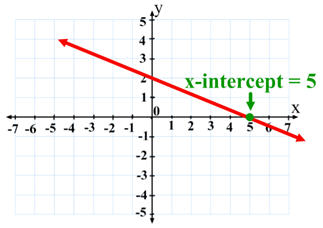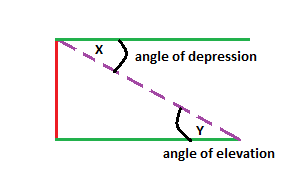## general form, slope y intercept, point slope,

general form:

if you ever get  a general form equation, you need to know that it tells you nothing about the equation and it is  pretty useless. general form will always have a zero at the end of the equation and the number at the start of the equation will always be positive if the number is not positive that means the equation is written in a wrong way. you also cant have any fractions in a general form equation. and example of a right general form equation is 5x-y+6=0, this equation is right because the first number is positive and there are no fractions seen in the equation and it all equals zero. a wrong general form equation would be  -8x+y-6/7=0, this is a wrong equation because the first number is a negative exponent and there is also a fraction in the equation.

point slope:
the equation point slop represents  the formula m(x,x) =y-y, you can use this type of formula when you are given two numbers and you need to put them into an equation, if you have the numbers (4,6) and (5,4) the equation would turn into 4(x-6)= y-6. the equations turned into this because we have the slope which is 4 because of the difference in the numbers and the slope will be IN front of the brackets, then you have to insert the x and the y numbers into the equation making it a -4 and – 6.

slope y intercept:

the equation slope y intercept is the y=mx+b equation. it tells you  both the slope and the y intercept. the mx part is the slope and the b is the y intercept, that means the you can graph a line on graphing paper using that formula.

## core competences

How does the artifact you selected demonstrate strengths & growth in the communication competency?

In what ways might you further develop your communication competency?

Self-Reflection

This class has helped me a lot with my mathematics skills and it also helped me grow and learn new skills and units that I did not know of last year. This class made me make new friends and made me talk to new people so they could help me if I am stuck on anything or I could help them if they needed my help from time to time. I am looking forward for next year to experience the same things that I experienced in math 10 but even better. I am looking forward to meeting new people and learning more mathematical skills that I could use in the future.

This class showed that math is not just fun and games it is a subject that is very important in the future and that I will be using it later in life. This class make work showed me that math is not as easy as you think, you need to work hard in this class and need to ask questions if you are stuck on something. It showed me that you can’t memorize math in your head or do equations in your head, you need to understand the formulas that you learn every day, and to practice for a test you need to write lots of notes so you can look back to what you did already

How does the artifact you selected demonstrate strengths & growth in the thinking competencies?

In what ways might you further develop your thinking competencies?

How does the artifact you selected demonstrate strengths & growth in the personal & social competencies?

In what ways might you further develop your personal & social competencies?

You will now attach and/or embed your self-assessment to the bottom of the blog post with the artifact you have chosen. You may choose to make this post private or public. After placing your artifact on a blog post, follow the instructions below.

1. Categories – Self-Assessment
2. Tags – Now tag your post using the tag that corresponds to the competency that you have written about. You can choose more than one. Please use lower case letters and be exact.
• #creativethinkingcc
• #communicationcc
• #criticalthinkingcc
• #socialresponsibilitycc
• #personalidentitycc
• #personalawarenesscc
3. Use the Add Document button located at the top of your post page and embed your self-assessment at the bottom of your blog post.
4. Publish1

## X-intercept & Y- intercept

The x-intercept is a point on the graph but on the other hand the y- intercept is a zero. the y- intercept is a point on the graph where the x becomes a zero. to calculate the x intercept you have to be using the formula (y-b), m=x, where the m equals the slope, and the y equals zero and the b equals the y-intercept. the y- intercept is the point which a linear equation crosses the y- axis on the x=0 plot point, the equation to determine both the slope and the y-intercept of a line is y=mx+b.## domain and range

The Range value means the difference between the lowest and the highest value in the diagram. But domain means the set on an input or argument values for which the function is defined. The domain and range of a function is all the possible values of the independent variable such as x, the rang of a function is all the possible values of the dependent variable AKA the Y. you can write domain and range in two ways, they are called the interval notation and the set notation.## ugly polynomials

The definition of ugly polynomials is that it is a mathematical expression of one or more algebraic terms each which consists of a constant multiplied by one or more then one variable raised to a nonnegative integer power. it is the sum of several terms that contains different powers of the same variable.## Rube Goldberg AKA The Dog Feedersteps:

step 1 : I am going to be pushing the ball to the water bottle that will be sitting on top of the shelf.

step 2: the water bottle is going to to fall down from the shelf and its going to hit the aluminum trays.

step3: the aluminum trays are going to give the onion that’s sitting on the edge vibration and its going to role down.

step 4: when the onion roles down it going to knock the cub with the potato down.

step5: when the cup with the potato falls down its weight is going to make the cup with the dog food tilt witch is going to cause the food to spill in the dog tray.

energy types:

the types if energy that I have used for this project was mechanical and gravitational energy. I used the mechanical when i pushed the soccer ball towards the water bottle that was sitting on the shelf, that caused the water bottle to fall down which that would be a gravitational energy. another mechanical energy was when the onion rolled down and pushed the cup with the potato which caused the cup to fall and that would be a gravitational  energy source.

Riverside CC’s Self Assessment Document (3)

## factoring in polynomials

The process of multiplying polynomials in order to return to unique string of polynomials of lesser degree whose product is the original polynomial. factoring polynomial is an action in which polynomial is represented as a product of simpler polynomials that cannot be further factored, you can factor polynomials by breaking down every term in the question into prime factors. this could expand the expression to look for factors that appear in every single term to determine the GCF.## Angle depression, Angle of evaluation

Angle depression: The angle of depression is one of the angles in a triangle. is is the angle that lays between a horizontal line from the observer and the line of sight to an object that is below the horizontal line. to calculate a depression angle you have to use the tangent formula, TAN= opposite side/adjacent.

Angle of evolution: The angle of evolution is the angle that is framed by the line of sight and the horizontal line from the observer and object vertical point. it can be calculated by knowing the value of the height and the distance of the object or the triangle. the formula for finding the angle of evolution depends on knowing the information to the measures of the opposite, hypotenuses, and the adjacent side of the right tringle.## Sine, Cosine, Tangent

those three words are one of the most important part of trigonometry.

1. sine: the word sine is know as SOH, sine is one of the three formulas in trigonometry. that formula is used to find on of the three angle in right side triangle. to find the angle that you looking for you need to divide the measurement that is opposite to the angle that your trying yo find.2. cosine: cosine that is also known as CAH is the adjacent side that is being divided by the the hypotenuse of the triangle to get the curtain  degree that you are looking for.3. tangent: tangent also know as TOA is the opposite side divided by the adjacent side. it is the only formula  beside the other two that does not need a hypotenuse as a denominator.